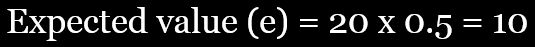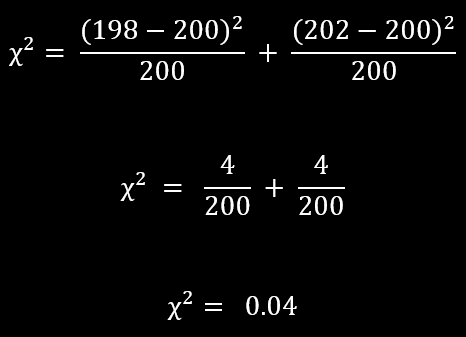top of page
Search
•Jolene Pappas

# How to test for evolution using the null hypothesis

Updated: Apr 27, 2021

Would you rather watch a video than read? Here is a video version of this post:

This post demonstrates how to test for evolution using the null hypothesis with data from a population genetics simulation. Evolution is the change in the inherited traits of a population over generations. It is important to note that evolution occurs in populations, not individuals. This activity specifically looks at microevolution, which examines changes in allele frequencies over relatively short periods of time, rather than macroevolution, which includes speciation and typically takes place over long periods of time.

The null hypothesis states that there is no significant difference between samples. "Significant" essentially means that the conclusion is probably not due to chance. Having said that, this isn’t a statistics tutorial, so understand that the use of statistical terms will be fairly basic. This post is primarily intended for AP Biology (and maybe APES) students and teachers, and perhaps intro level college biology students.

For testing evolution, the samples being compared are the starting and ending allele frequencies for a specific gene during a given time period. Note that only a single gene is being tested. If the frequencies change over time, then evolution is occurring. If not, then the population is at equilibrium.

In this context, there are two possible outcomes when testing the null hypothesis; rejecting the hypothesis and failing to reject the hypothesis. If the null hypothesis is rejected there is probably a significant difference between the samples. This means that ending frequencies are not the same as the starting frequencies, so evolution occurred during the time period. If the null hypothesis is not rejected then there probably isn't a significant differences between the starting and ending frequencies, so evolution did not occur for that gene in that time period.

For this example, we’ll use chi-squared (also written as chi-square) as a statistical test. To use the null hypothesis to test for evolution, our expected values (e) will be the starting number of alleles and the observed values (o) will be the final allele numbers.Chi-squared equation

To get some sample data, we'll use a run of the population genetics simulation. A gene for color in this fictional population has two alleles; red and blue. There are three possible phenotypes; red and blue are homozygotes and the heterozygotes are purple. Check out this post for additional information about the population genetics simulation. For this trial run, the final frequency for the red allele is 0.8, and the blue allele is 0.2. These are the numbers we’ll be using to calculate the observed values for the chi squared test.Both alleles started at a frequency of 0.5. After 20 generations the red allele is 0.8 and the blue is 0.2.

If evolution did NOT occur (the null hypothesis) then the final number of red and blue alleles will be close to the same as the starting number of red and blue alleles. Our expected numbers (e) are be based on the starting frequency. In this case, the starting frequency for the red allele was set to 0.5, so the frequency for both alleles is 0.5.

To find the number of alleles for our expected values, we use the total number of alleles and the ending frequency. The population size is set to 10 individuals, and each of those individuals have two copies of each gene. Therefore, the total number of alleles is 20.Total number of alleles in a population of 10

To calculate the number of alleles, multiply the frequency by the total number of alleles. In this case, the expected value (e) is 10 for both the red and blue alleles. To get the observed values (o), multiply the final frequencies by 20. The final frequency for red is 0.8, so the final number of red alleles is 16. The final frequency for the blue allele is 0.2, so the final number of blue alleles is 4.expected value calculationobserved value calculations

Next, plug the expected (e) and observed (o) values into the chi-squared equation for both red and blue.Chi-squared calculation

Our chi-squared value for this trial is 7.2. This value by itself doesn’t tell us anything, which is where the critical value table comes in. To find the critical value on the table we need to know two pieces of information; the degrees of freedom and the p-value.

The degrees of freedom is the number of categories minus 1. We have two categories, red and blue, so we have one degree of freedom. The p-value is the probability that the results could be due to chance. There is a lot that can be said about p-values and their use in scientific statistical tests, but as this isn’t a stats tutorial, I’m not going into it.

In this context, we are going to use a p-value of 0.05, which is typically what will be used in most high school biology situations. Looking at the chi-square table, the 1 degree of freedom column and the 0.05 p-value row give us a critical value of 3.84. To interpret our results we compare our chi-squared value to the critical value. If the chi-squared value is greater than the critical value the null hypothesis is rejected and we conclude that there is a difference between the samples.

If the Chi-squared value is less than, or equal to, the critical value, then we fail to reject the null hypothesis and there probably is not a difference between our samples.

For our example, the chi-squared value is 7.2 and the critical value is 3.83, so the null hypothesis (that the observed and expected values are the same) is rejected. Based on that, we conclude that evolution did occur for this gene in the time frame studied.

Let's look at another set of data. This time the population size was set to 200, but the other parameters are the same as before. For this run, the final allele population is 0.495 for the red and 0.505 for the blue. This time, we have a total of 400 alleles in the population. Starting out we had an even split again, so the allele numbers are 200 red and 200 blue.Trial run of the population genetics simulation with a population of 200

We set the red allele starting frequency to 0.5, so the allele numbers are 200 red and 200 blue. Because we’re testing the null hypothesis, which means there isn’t any change over time, our expected values will be 200. Because we’re testing the null hypothesis, which means there isn’t any change over time, our expected values will be 200. For the red allele our observed value is 198 and for blue it is 202.Calculation of expected and observed values

Plug these values into the chi-squared equation to get a value of 0.04. This is less than the critical value of 3.84, so we fail to reject the null hypothesis. This means we can conclude that there wasn’t a significant change in the allele frequencies and so the color gene in this population is probably at genetic equilibrium.Chi-squared calculation

Keep in mind that this approach only tests the final frequencies, so it doesn’t necessarily account for fluctuations that are occurring over the course of the tested time period. Also, these examples only looked at allele numbers, but you could also try using the test for phenotypes. Finally, if you are using this to test if certain parameters are likely to lead to evolution, remember that one benefit of simulations is the ability to run many trials relatively quickly. The more trials you run, the more clear of a picture you can get for the range of possible results.